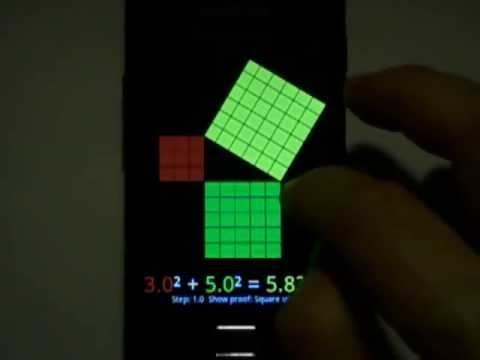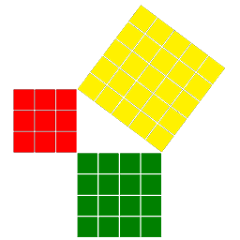# Touch Pythagoras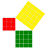5K+Everyone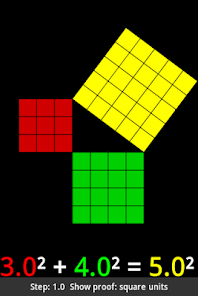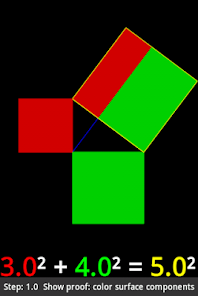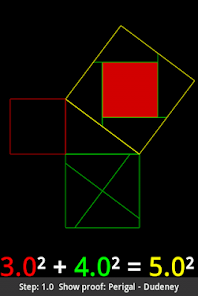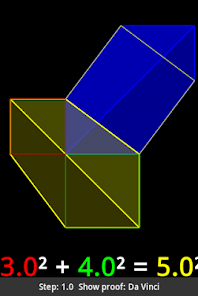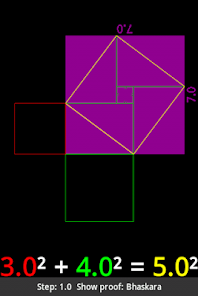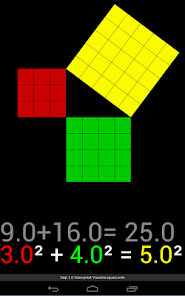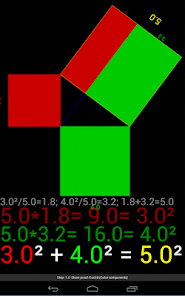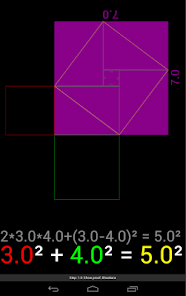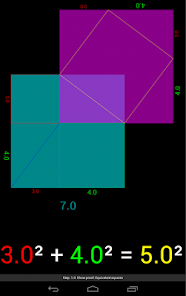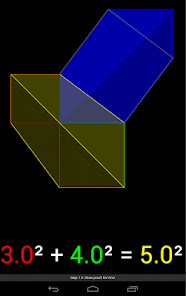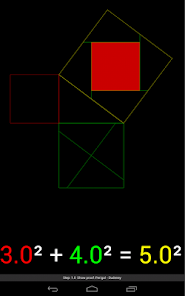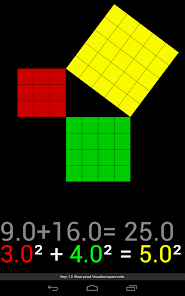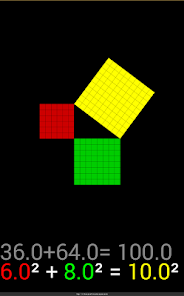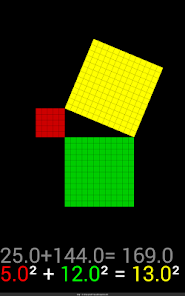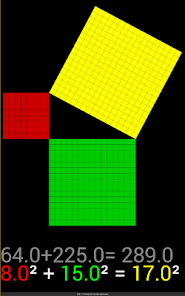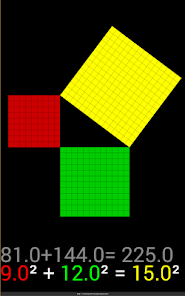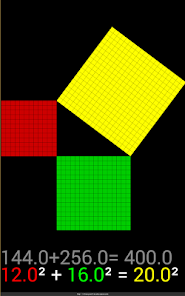The Pythagorean Theorem Interactive: a^2 + b^2 = c^2

App :
change the lengths of the legs (dragging).
change the length of the hypotenuse with two fingers.
zoom (pinch zoom) and rotate the figure (dragging).

There are 6 ways to view the Pythagorean theorem.
- Unit surfaces.
- Two equivalent square containing the same surface.
- The square for each leg in the square of the hypotenuse (Euclid)
- Pingi - Dudeney proof.
- Da Vinci.

Change the precision of the lengths. (In the contextual menu)

This application is also a small laboratory to investigate about the Pythagorean Theorem:
For example, you can experiment easily, looking for the exact solutions of the Pythagorean Theorem:
3² + 4² = 5² is not the only exact solution:

Below 21, there are 3 primitive triples:
3² + 4² = 5²
5² + 12² = 13²
6² + 8² = 10² (Not a true primitive result: Multiple of 3,4,5)
8² + 15² = 17²
9² + 12² = 15² (Not a true primitive result: Multiple of 3,4,5)
12² + 16² = 20² (Not a true primitive result: Multiple of 3,4,5)
Likewise it is also possible to find the solutions below 31 (11 solutions in all: but only 5 primitives)
Or solutions below 101 (52 solutions in all: but only 16 primitives)

More primitive pythagorean triples:
9² + 40² = 41²
11² + 60² = 61²
12² + 35² = 37²
13² + 84² = 85²
15² + 112² = 113²
16² + 63² = 65²
17² + 144² = 145²
19² + 180² = 181²
20² + 21² = 29²
20² + 99² = 101²
24² + 143² = 145²
28² + 45² = 53²
33² + 56² = 65²
36² + 77² = 85²
39² + 80² = 89²
44² + 117² = 125²
48² + 55² = 73²
51² + 140² = 149²
52² + 165² = 173²
57² + 176² = 185²
60² + 91² = 109²
65² + 72² = 97²
85² + 132² = 157²
88² + 105² = 137²
95² + 168² = 193²
104² + 153² = 185²
119² + 120² = 169²
133² + 156² = 205²
140² + 171² = 221²

Touch Pythagoras is a part of the collection Touch Math Apps
Updated on
Oct 8, 2020

## Data safety

Safety starts with understanding how developers collect and share your data. Data privacy and security practices may vary based on your use, region, and age. The developer provided this information and may update it over time.No data shared with third parties